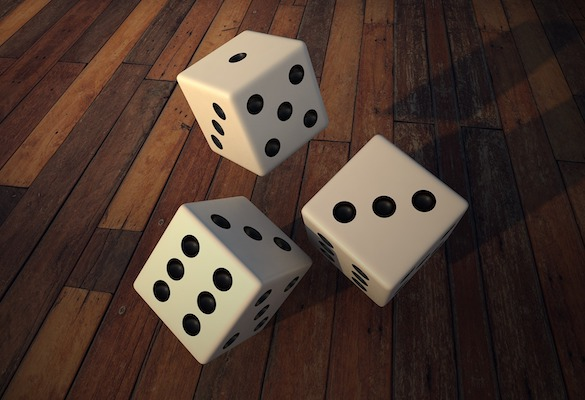## How to Create a Random Whole Number in JavaScript

##### In this tutorial we explain how to generate a random whole number using JavaScript. Scroll to the end for the TL;DR if you just want the line itself!#### Need a new t-shirt?

Creating random whole numbers in programming is a relatively common task. Once you know how to do so it is quick and easy… just one line of code. In this quick tutorial we will show you how. We will build up the code to make sure you understand what each part means.

To help with context, let’s say we want to create a random whole number that is greater or equal to 1 and less than or equal to 20. We want to store the number as a variable called number.

## Step 1: generating a random number

JavaScript has a simple method for creating a random number. “Great! This is going to be easy then!” you might think. Whilst you’d be kinda right (it is only a single line we’re building up to after all), it’s also not quite as simple as that. The random number that is returned will be greater than or equal to 0 and less than 1. Not great when we’re trying to generate a whole number between 1 and 20! It is where we have to start though.

```const number = Math.random();
```

Math” is an Object given to us by JavaScript. It has a number of built in Methods which we can use. One of the methods is random(). As a method is basically a type of function, it is followed by the parentheses.

What the line above is saying is,

“Make me a variable called number and make its value a random number between 0 (inclusive) and 1 (exclusive).”

## Step 2: multiply by 20

The first thing we need to do is to increase the size of our random number. To do so we multiply it by the highest number we are looking to achieve. In our example we want our maximum number to be 20 so that is what we will multiply by. That means the next iteration of our line of code is:

```const number = Math.random()*20;
```

What the line above is saying is,

“Make me a variable called number. Calculate its value for me by taking a random number between 0 (inclusive) and 1 (exclusive) and multiplying that number by 20.”

When we do that, we are creating a random number between 0 (inclusive) and 20 (exclusive). That random number will likely have a large number of decimal places after it. Whilst we’re closer to our end goal we still have a bit left to do.

## Step 3: remove the decimal places

Our random number (number) can now be defined as follows:

0 <= number < 20 with any number of decimal places.

However that is not good enough as our end goal is:

1 <= number <= 20 with zero decimal places

Removing the decimal places is not too tricky as, again, JavaScript has us covered with its built-in tools.

```const number = Math.trunc(Math.random()*20);
```

As with equations in mathematics, JavaScript will calculate the value of number by carrying out the calculation in parentheses first and then doing the next part.

So Math.random()*20 will be calculated first. Math.trunc() will then be done on the resulting number. As with Step 1, Math is the object and, in this case, trunc() is the method.

What the above line is saying is,

“Make me a variable called number. Calculate its value for me by making a random number between 0 (inclusive) and 20 (exclusive) and then truncate it to remove the decimal points.”

This line will produce an integer (a whole number) between 0 (inclusive) and 19 (inclusive). Nearly there!

## Step 4: elevate the range by 1

This is probably the easiest step of the building of our line of code. Maybe even one that some of you may have been able to work out yourselves. Don’t worry if you haven’t though… there has been a lot to take in and probably new things for you to see and learn. The fact you have got through to this stage of the tutorial means you’re doing all the right things to become a great JavaScript Developer.

The previous step got us a whole number between 0 and 19. We need that to be 1 and 20 so we simply add 1 as below.

```const number = Math.trunc(Math.random()*20) + 1;
```

What the above line is saying is,

“Make me a variable called number. Calculate its value for me by making a random integer between 0 (inclusive) and 19 (inclusive) then add 1 to that random number.”

The lowest random number generated before adding the 1 will be 0 which means our lowest number will be 1. The highest random number before adding the 1 is 19 which means our highest number will be 20. We won’t have any decimal places so we are all done!

## TL;DR

To make a random whole number between 1 and 20 use the following line of code:

```const number = Math.trunc(Math.random()*20) + 1;
```